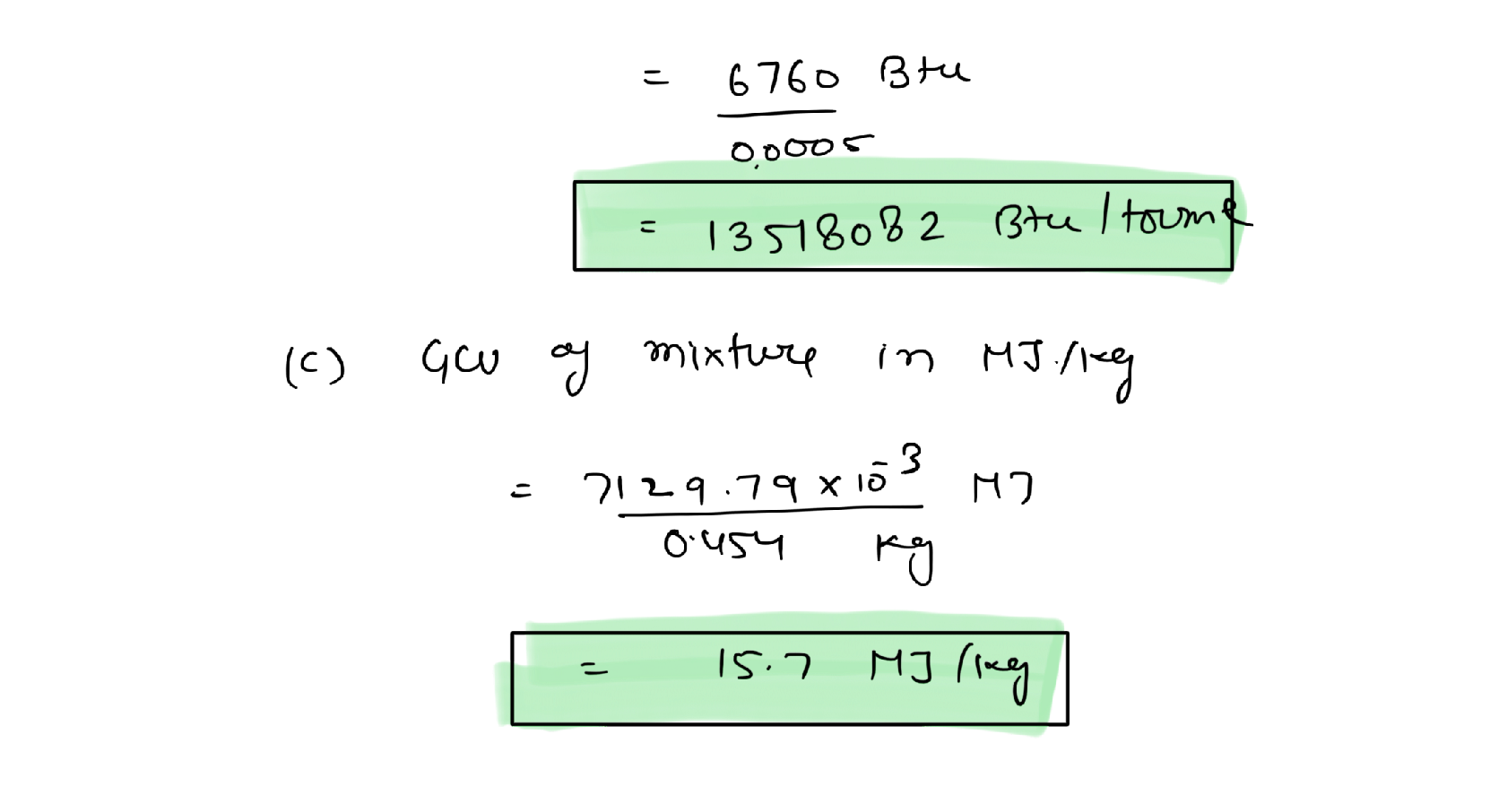Question

Air Pollution

2. From the elemental analysis, a waste mixture contains 15% by weight of ash, and the other portion of the mixture can be represented by a chemical formula of C600h1000O400N10s

(a) Use Dulong's formula to estimate the heating value of this waste mixture in Btu/lb,assuming the ash has no heating value.

(b) Convert the heating value of the heat mixture into million Btu/ton

(c) Use the conversion factors between Btu and kJ and between Ib and kg to convert the heating value of the waste mixture into MJ/kgVerified### Question 28908Air Pollution

5. Briefly introduce Puente Hills Landfills and its closure in 150 - 200 words.

### Question 28907Air Pollution

Assuming air contains 20% by volume 0; and 80% N3, for combustion of 100 standard cubic feet per minute (sefm) of methane (CH.4) in air.
\mathrm{CH}_{4}+2 \mathrm{O}_{2} \rightarrow \mathrm{CO}_{2}+2 \mathrm{H}_{0} \mathrm{O}
The stoichiometric amount of O; needed for a complete combustion (in scfm)
(b) The stoichiometric amount of air needed for a complete combustion (in sefm)
(d) The A/F ratio (in a mass/mass ratio)
(c) The stoichiometric mixture by volume (in % methane in the stoichiometric mixture)

### Question 28906Air Pollution

3. List two active MSWS that are closest to CSU Fullerton. List three closed MSWS that areclosest to CSU Fullerton

### Question 28905Air Pollution

2. From the elemental analysis, a waste mixture contains 15% by weight of ash, and the other portion of the mixture can be represented by a chemical formula of C600h1000O400N10s
(a) Use Dulong's formula to estimate the heating value of this waste mixture in Btu/lb,assuming the ash has no heating value.
(c) Use the conversion factors between Btu and kJ and between Ib and kg to convert the heating value of the waste mixture into MJ/kg
(b) Convert the heating value of the heat mixture into million Btu/ton

### Question 28904Air Pollution

1. Which of the following media contains the largest amount of toluene, CeHsCH3 (show your calculations)?
(a) 1 million gallons of water containing 5 ppm of toluene
(b) 100 cubic meters of soils (bulk density = 1.8 g/cu. cm) with 5 ppm of toluene
(c) An empty warehouse (30 m x 15 m x 10 m) with 5 ppmV toluene in air (P = 1 atm and T20 °C).

### Submit query